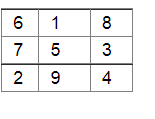# complete the magic square given below,

Question:

complete the magic square given below, so that the sum of the numbers in each row or in each column or along each diagonal is 15.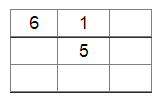Solution:

Taking the diagonal that starts with 6:

$6+5+x=15 \Rightarrow x=4$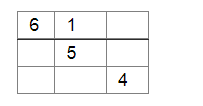Now, taking the first row:

$6+1+x=15 \Rightarrow x=8$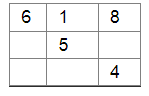Taking the last column:

$8+x+4=15 \Rightarrow x=3$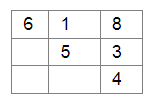Taking the second column:

$1+5+x=15 \Rightarrow x=9$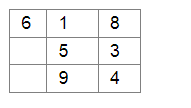Taking the second row:

$x+5+3=15 \Rightarrow x=7$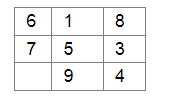Taking the diagonal that begins with 8:

$8+5+x=15 \Rightarrow x=2$